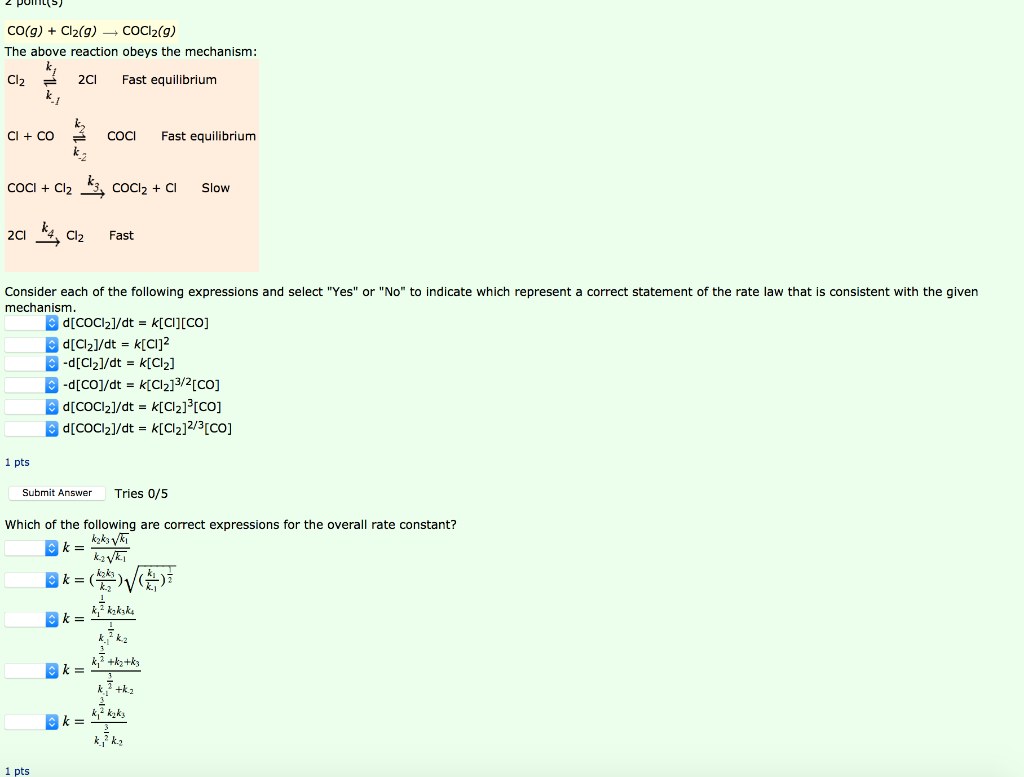# Question Solved1 Answerundefined POMLS CO(g) + Cl2(9) COCI2(9) The above reaction obeys the mechanism: Cl2 2CI Fast equilibrium ki Cl + CO COCI Fast equilibrium Slow COCI + Cl2 kz, COCl2 + C1 201 kg, Cl2 Fast Consider each of the following expressions and select "Yes" or "No" to indicate which represent a correct statement of the rate law that is consistent with the given mechanism. d[COC12]/dt = K[C][CO] [Cl]/dt = K[CI] -d[Cl2]/dt = K[Cl] -d[Co]/dt = K[Cl2]3/2[co] d[CoCl2]/dt = K[Cl2][CO] d[COCI2]/dt = k[Cl2]2/3[CO] 1 pts Submit Answer Tries 0/5 Which of the following are correct expressions for the overall rate constant? k= kaks ki kaki k= ka kzkzke k k= k, 7+ka+k; +k2 k= k, k2 1 ptsundefined

Transcribed Image Text: POMLS CO(g) + Cl2(9) COCI2(9) The above reaction obeys the mechanism: Cl2 2CI Fast equilibrium ki Cl + CO COCI Fast equilibrium Slow COCI + Cl2 kz, COCl2 + C1 201 kg, Cl2 Fast Consider each of the following expressions and select "Yes" or "No" to indicate which represent a correct statement of the rate law that is consistent with the given mechanism. d[COC12]/dt = K[C][CO] [Cl]/dt = K[CI] -d[Cl2]/dt = K[Cl] -d[Co]/dt = K[Cl2]3/2[co] d[CoCl2]/dt = K[Cl2][CO] d[COCI2]/dt = k[Cl2]2/3[CO] 1 pts Submit Answer Tries 0/5 Which of the following are correct expressions for the overall rate constant? k= kaks ki kaki k= ka kzkzke k k= k, 7+ka+k; +k2 k= k, k2 1 pts
More
Transcribed Image Text: POMLS CO(g) + Cl2(9) COCI2(9) The above reaction obeys the mechanism: Cl2 2CI Fast equilibrium ki Cl + CO COCI Fast equilibrium Slow COCI + Cl2 kz, COCl2 + C1 201 kg, Cl2 Fast Consider each of the following expressions and select "Yes" or "No" to indicate which represent a correct statement of the rate law that is consistent with the given mechanism. d[COC12]/dt = K[C][CO] [Cl]/dt = K[CI] -d[Cl2]/dt = K[Cl] -d[Co]/dt = K[Cl2]3/2[co] d[CoCl2]/dt = K[Cl2][CO] d[COCI2]/dt = k[Cl2]2/3[CO] 1 pts Submit Answer Tries 0/5 Which of the following are correct expressions for the overall rate constant? k= kaks ki kaki k= ka kzkzke k k= k, 7+ka+k; +k2 k= k, k2 1 pts